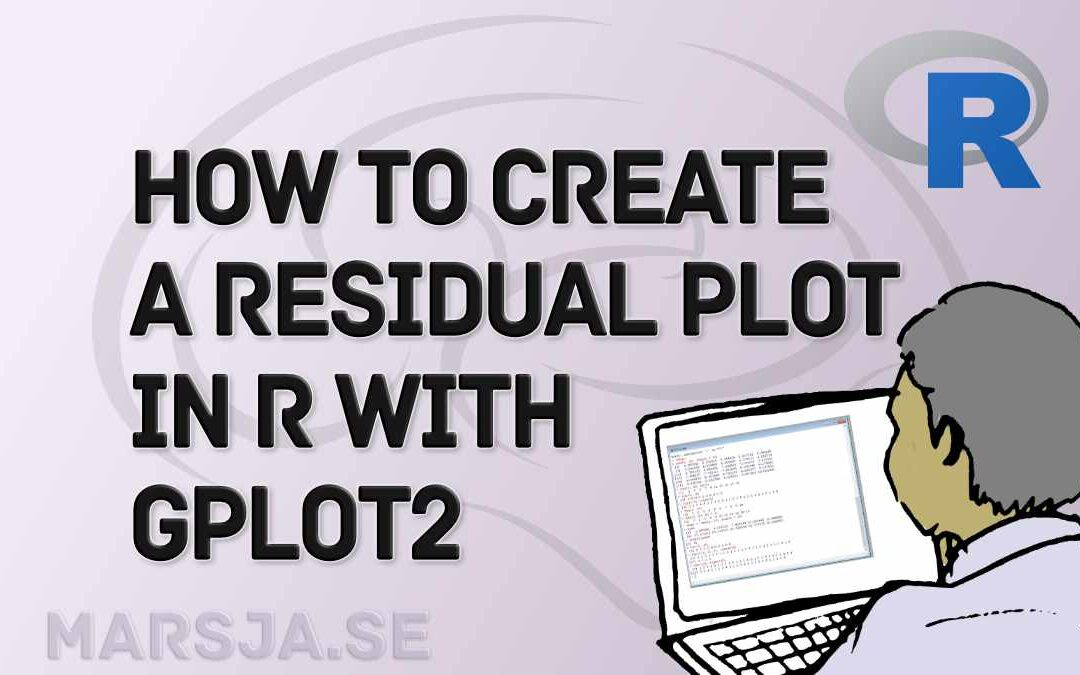## How to Make a Residual Plot in R & Interpret Them using ggplot2

In this post, you will learn how to make a residual plot in R. Moreover, you will learn when to use different residual plots and how to interpret them. Residual plots are a graphical tool that can evaluate the quality of a regression model. They are handy for...## How to do a Kruskal-Wallis Test in R

This post will teach you how to carry out the Kruskal-Wallis test in R. The Kruskal-Wallis test is a non-parametric statistical test used to determine whether there is a significant difference in the median values of two or more independent groups. This method is used...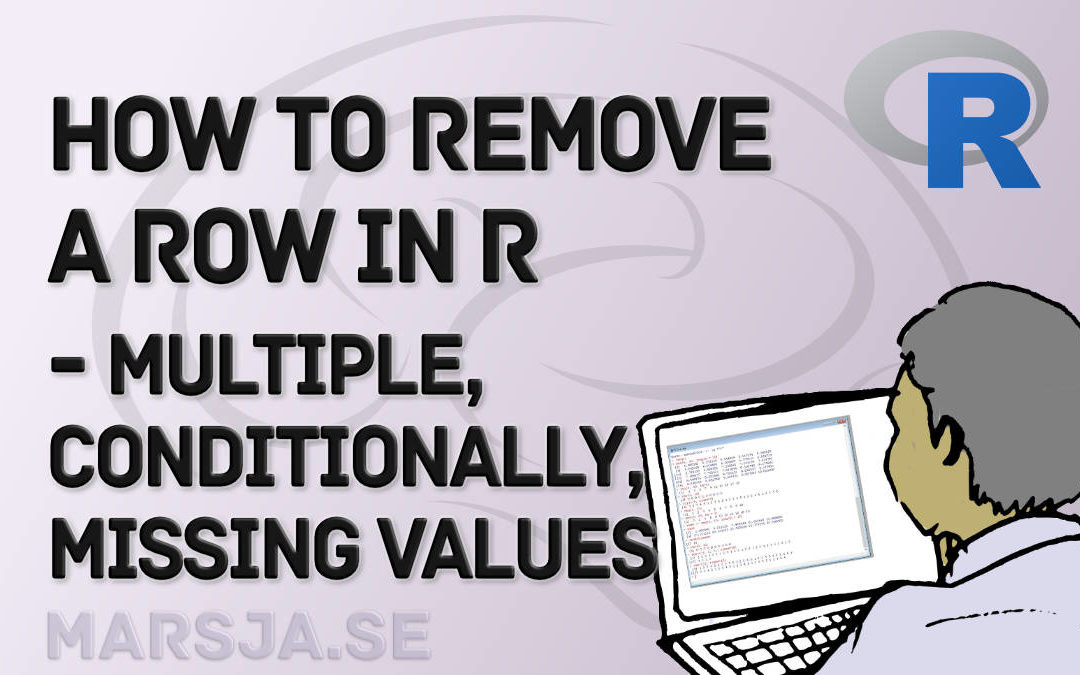## How to Remove/Delete a Row in R – Rows with NA, Conditions, Duplicated

In this tutorial, you will 1) learn how to delete a row in R and 2) how to remove multiple rows in R. Of course, R being a universal programming language, there are many different options when we need to delete a row. For example, we can use the subset() function to...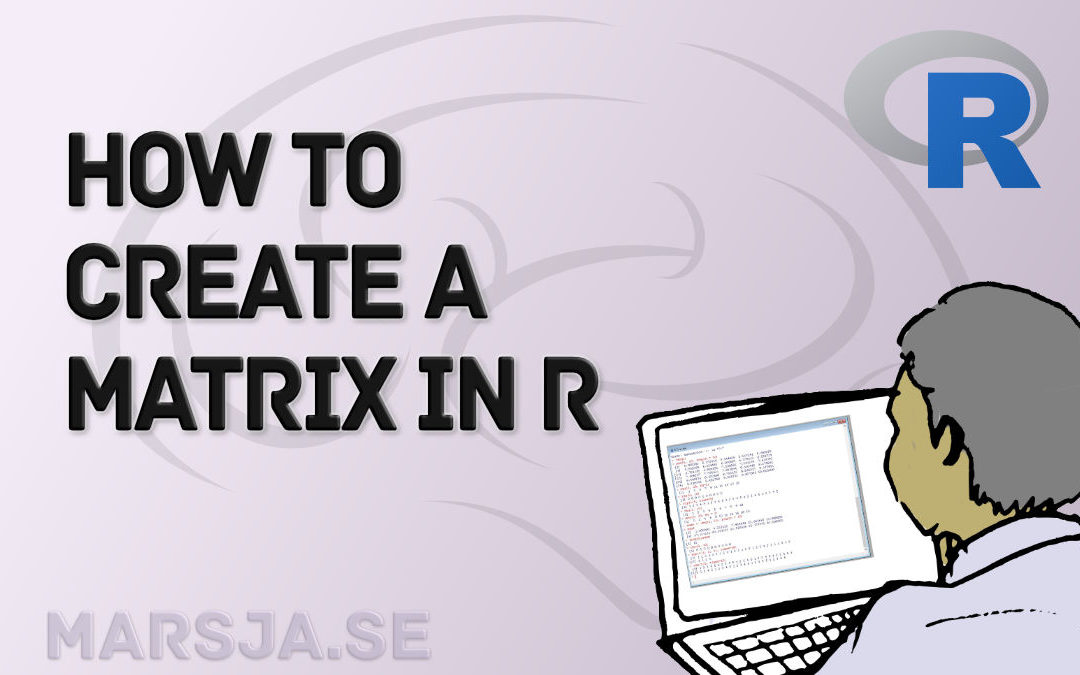## How to Create a Matrix in R with Examples – empty, zeros

In this short tutorial, you will learn how to create a matrix in R. We will use the matrix() function, among two other functions, for this aim. Specifically, we will go into the details of this function as this will enable us to e.g. name the columns and rows in the...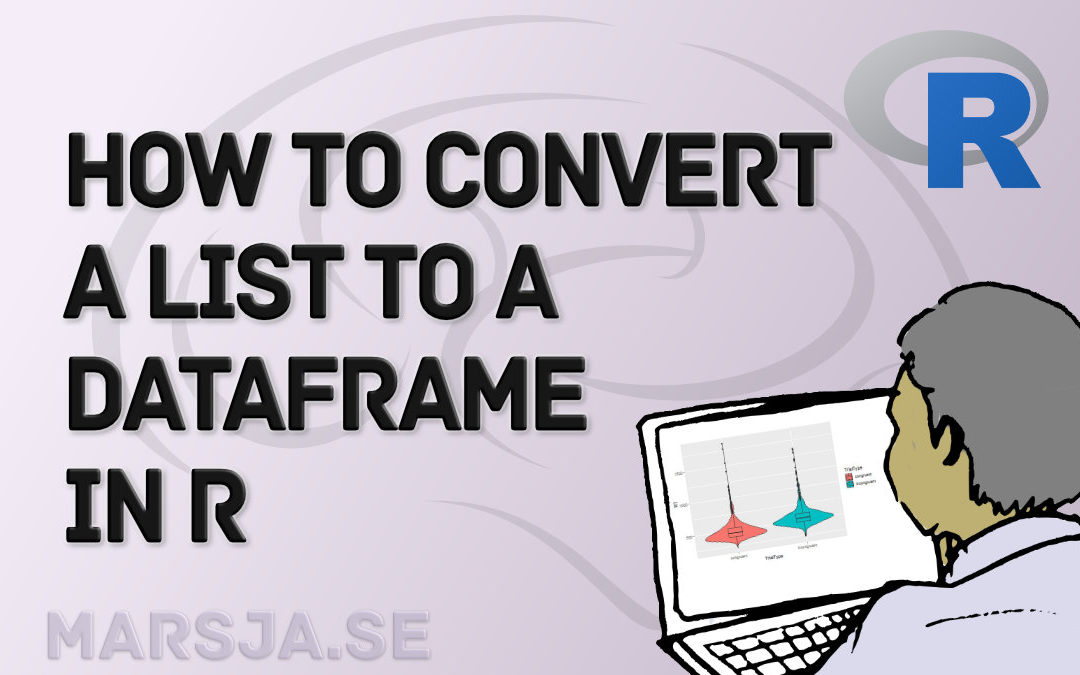## How to Convert a List to a Dataframe in R – dplyr

In this short tutorial, you will learn how to convert a list to a dataframe in R. To know how to convert lists to dataframes may be useful when you, for example, get your data from a source and they end up in a list of e.g. vectors. Here’s an example code template...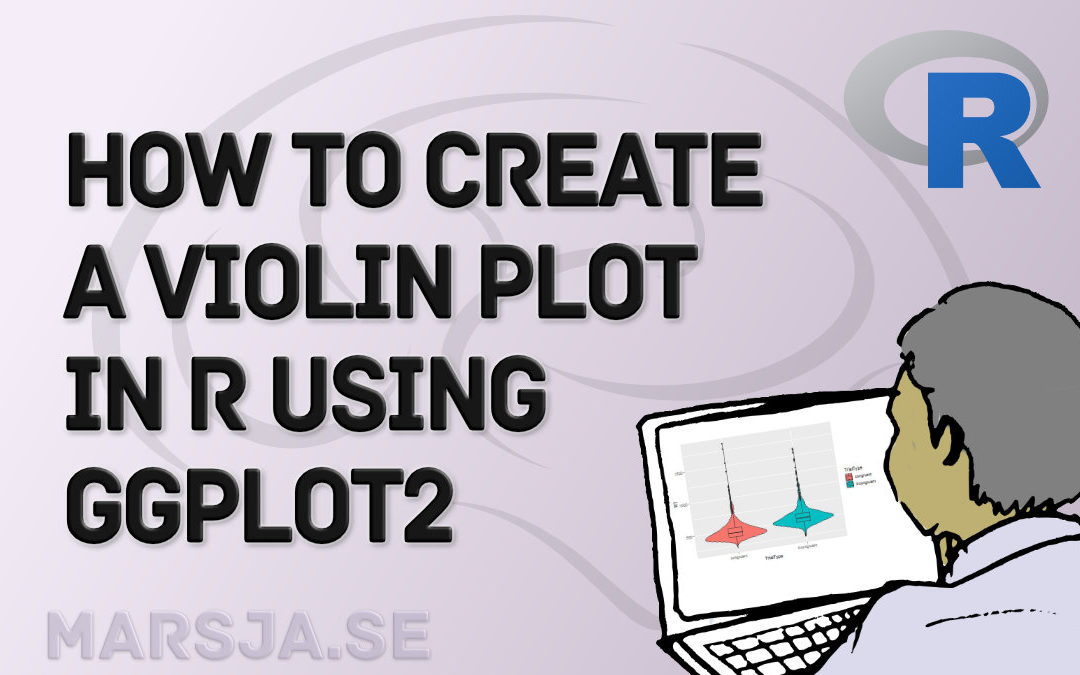## How to Create a Violin plot in R with ggplot2 and Customize it

This data visualization tutorial will teach us how to make a violin plot in R using ggplot2. We can use several techniques to visualize data (see, for example, the Python-related post “9 Data Visualization Techniques You Should Learn in Python”) to...